## Monday, January 23, 2017

### Azure Machine Learning: Model Evaluation using ROC (Receiver Operating Characteristic)

Today, we're going to continue looking at Sample 3: Cross Validation for Binary Classification Adult Dataset in Azure Machine Learning.  In the four previous posts, we looked at the Two-Class Averaged PerceptronTwo-Class Boosted Decision TreeTwo-Class Logistic Regression and Two-Class Support Vector Machine algorithms.

In all of these posts, we used a simple contingency table to determine the accuracy of the model.  However, accuracy is only one of a number of different ways to determine the "goodness" of a model.  Now, we need to expand our evaluation to include the Evaluate Model module.  Specifically, we'll be looking at the ROC (Receiver Operating Characteristic) tab.  Let's start by refreshing our memory on the data set.Adult Census Income Binary Classification Dataset (Visualize)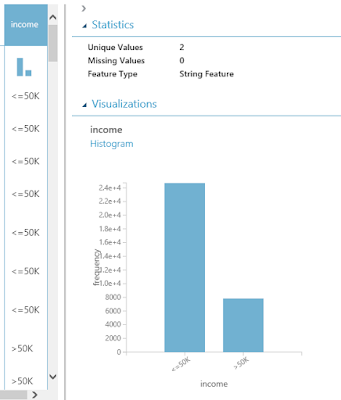Adult Census Income Binary Classification Dataset (Visualize) (Income)
This dataset contains the demographic information about a group of individuals.  We see the standard information such as Race, Education, Martial Status, etc.  Also, we see an "Income" variable at the end.  This variable takes two values, "<=50k" and ">50k", with the majority of the observations falling into the smaller bucket.  The goal of this experiment is to predict "Income" by using the other variables.

Utilizing some of the techniques we learned in the previous posts, we'll start by using the "Tune Model Hyperparameters" module to select the best sets of parameters for each of the four models we're considering.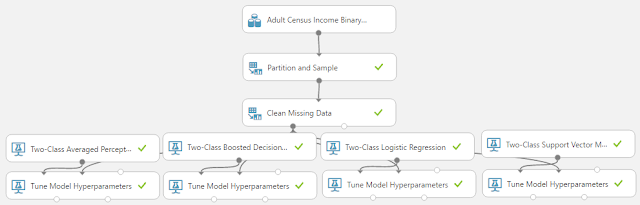Experiment (Tune Model Hyperparameters)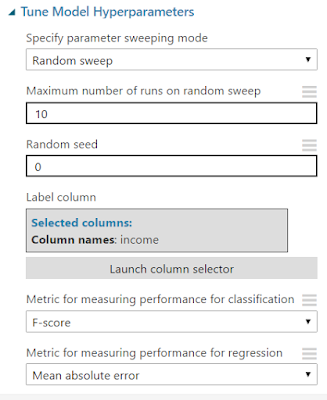Tune Model Hyperparameters
As you can see, we are doing a Random Sweep of 10 runs measuring F-scores.  One of the interesting things about the "Tune Model Hyperparameters" module is that it not only outputs the results from the Tuning, it also outputs the Trained Model, which we can feed directly into the "Score Model Module".

At this point, we have two options.  For simplicity's sake, we could simply train the models using the entire data set, then score those same records.  However, that's considered bad practice as it encourages Overfitting.  So, let's use the "Split Data" module to Train our models with 70% of the dataset and use the remaining 30% for our evaluation.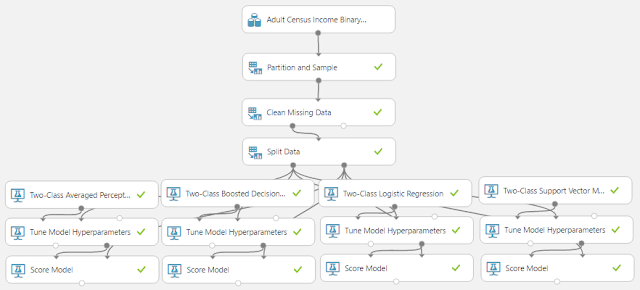Experiment (Score Model)Split Data
Looking back at the "Income" histogram at the beginning of the post, we can see that a large majority of the observations fall into the "<=50k" category.  This creates an issue known as an "imbalanced class".  This means that there is a possibility that one of our samples will contain a very small proportion of ">50k" while the other sample contains a very large proportion.  This could cause significant bias in our final model.  Therefore, it's safe to use a Stratified Sample.  In this case, the Stratification Key Column will be "Income".  Simply put, this will cause the algorithm to take a 70% sample from the "<=50k" category and a 70% sample from the ">50k" category.  Then, it will combine these together to make the complete 70% sample.  This guarantees that our samples have the same distribution as our complete dataset, but only as "Income" is concerned.  There may still be bias on other variables.  Alas, that's not the focus of this post.  Let's move on to the "Evaluate Model" module.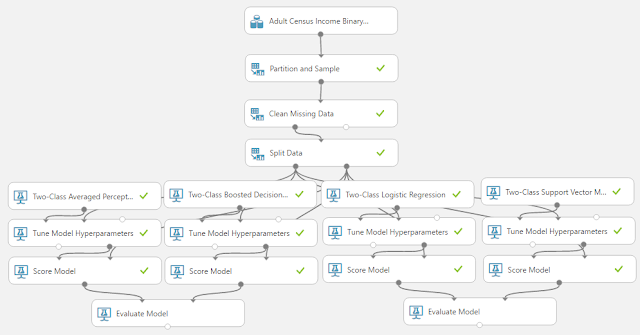Experiment (Evaluate Model)
We chose not to show you the parameters for the "Score Model" and "Evaluate Model" modules because they are trivial for the former and non-existent for the latter.  What is important is recognizing what the inputs are for the "Evaluate Model" module.  The "Evaluate Model" module is designed to compare two sets of scored data.  This means that we need to consider how we're going to handle our four sets of scored data.  If we wanted to be extremely thorough, we could use six modules to connect every set of scored data to every other set of scored data.  This may be helpful in case there are any cases where one model is good is some areas and weak in others.  For our case, it's just as easy to compare them as pairs, then compare the winners from those pairs.  Let's take a look at the "ROC" tab of the "Evaluate Model" visualization.  Given the size of the results, we'll have to look at it piece-by-piece.ROC Tab
In the top left corner of the visualization, you will see three labels for "ROC", "Precision/Recall", and "Lift".  For this post, we'll be covering the ROC tab, which you can find by clicking on the ROC button in the top left, although it should be highlighted by default.ROC Experiment View
If you scroll down a little, you will see a view of the Experiment on the right side of the visualization.  This might not seem too handy at first.  However, you should take note of which dataset is coming in the left and right sides.  In our case, this would be Averaged Perceptron on the left and Boosted Decision Tree on the right.ROC Chart
On the left side of the visualization, you will find the ROC Curve.  This chart will tell you how "accurate" your model is at predicting.  We like to think of the ROC Curve as follows: "If we want a True Positive Rate of Y, we must be willing to accept a False Positive Rate of X".  Therefore, a "better" model would have a higher True Positive Rate for the same False Positive Rate.  Conversely, we could say that a "better" model would have a lower False Positive Rate for the same True Positive Rate.  In the end, True Positive predictions are a good thing and should be maximized.  Moreover, False Positive predictions are a bad thing and should be minimized.  Therefore, we are looking for a curve that travels as close to the top-left as possible.  In this case, we can see the "Scored dataset to compare" is a "better" model.  In order to find out which model that is, we have to look over at the ROC Experiment View on the right side of the visualization.  We can see that the "Scored Dataset" (i.e. the left input) is the Averaged Perceptron, while the "Scored Dataset To Compare" (i.e. the right input) is the Boosted Decision Tree.  Therefore, the Boosted Decision Tree is the more accurate model according to the ROC Curve.

As an added note, there is a grey diagonal line that goes across this chart.  That's the "Random Guess" line.  It follows a line for 50% probability of guessing correctly, just like if we flipped a coin.  If we find that our model dips below that line, then that means our model is worse than random guessing.  In that case, we should seriously reconsider a different model.  If the model is always significantly below that line, then we can simply swap our predictions (True becomes False, False becomes True) to create a good model.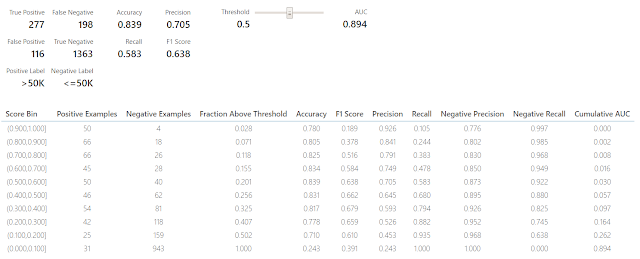Threshold and Evaluation Tables
If we scroll down to the bottom of the visualization, we can see some tables.  We're not sure what these tables are called, so we've taken to calling them the Threshold table (top table with slider) and the Evaluation table (bottom table).  These tables are interesting in their own right and will be covered in a later post.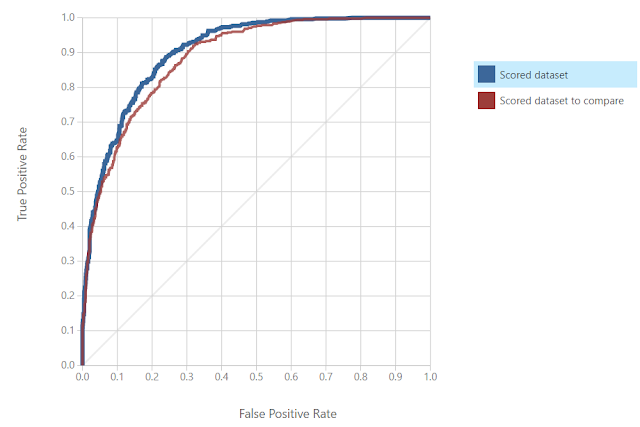ROC Curve 2
Look at the ROC Curve for the other "Evaluate Model" visualization, we can see that the Logistic Regression model is slightly more accurate than the Support Vector Machine.  Now, let's create a final "Evaluate Model" module to compare the winner from the first ROC analysis (Boosted Decision Tree) to the winner from the second ROC analysis (Logistic Regression).ROC Curve (Final)
We can see that the ROC Curve has determined that the Boosted Decision Tree is the most accurate model out of the four.  This wasn't a surprise to use because we did a very similar analysis using contingency tables in the previous four posts.  However, model evaluation is all about gathering an abundance of evidence in order to make the best decision possible.  Stay tuned for later posts where we'll go over more information in the "Evaluate Model" visualization.  Thanks for reading.  We hope you found this informative.

Data Scientist
Valorem
@BreakingBI
llewellyn.wb@gmail.com

## Monday, January 2, 2017

### Azure Machine Learning: Classification Using Two-Class Support Vector Machine

Today, we're going to continue looking at Sample 3: Cross Validation for Binary Classification Adult Dataset in Azure Machine Learning.  In the three previous posts, we looked at the Two-Class Averaged PerceptronTwo-Class Boosted Decision Tree and Two-Class Logistic Regression algorithms.  The final algorithm in the experiment is Two-Class Support Vector Machine.  Let's start by refreshing our memory on the data set.Adult Census Income Binary Classification Dataset (Visualize)Adult Census Income Binary Classification Dataset (Visualize) (Income)

This dataset contains the demographic information about a group of individuals.  We see the standard information such as Race, Education, Martial Status, etc.  Also, we see an "Income" variable at the end.  This variable takes two values, "<=50k" and ">50k", with the majority of the observations falling into the smaller bucket.  The goal of this experiment is to predict "Income" by using the other variables.  Let's take a look at the Two-Class Support Vector Machine algorithm.Two-Class Support Vector Machine
The Two-Class Support Vector Machine algorithm attempts to define a boundary between the two sets of points such that all of the points of one type fall on one side and all of the points of the other type fall on the other side.  More specifically, it attempts to define the boundary where the distance between the two sets of points is at its largest.  This is a relatively simple concept to imagine in two dimensions, but gets complex as your number of factors increases and the relationship between the factors becomes more complex.  Here's a picture that tells the story pretty nicely.Support Vector Machine
Let's take a look at the parameters involved in this algorithm.  First, we need to define the "Number of Iterations".  Simply put, more iterations means that the algorithm is less likely to get stuck in an awkward portion of data.  Therefore, it increases the accuracy of your predictions.  Unfortunately, this also means that the algorithm will take longer to train.

The "Lambda" parameter allows us to tell Azure ML how complex we want our model to be.  The larger we make our "Lambda", the less complex our model will end up being.

The "Normalize Features" parameter will replace all of our values with "Normalized" values.  This is accomplished by taking each value, subtracting the mean of all the values in the column, then dividing the result by the standard deviation of all the values in the column.  This has the effect of making every column have a mean of 0 and a standard deviation of 1.  Since the algorithm chooses a boundary based on distance between points, it is imperative that your values be normalized.  Otherwise, you may have a single (or small subset) of factors that dominate the selection process because they have very large values, and therefore very large distances.  If we wanted to have certain factors play a larger role in the selection process for some type of technical or business reason, then we could forego this option.  However, that situation would be better handled by multiplying the normalized factors by our own custom sets of "weights" using a separate module.

The "Project to Unit Sphere" parameter allows us to normalize our set of output "Coefficients" as well.  In our testing, this didn't seem to have any impact on the predictability of the model.  However, it may be useful if we need to use the coefficients as inputs to some other type of model which would require them to be normalized.  If anyone knows of any other uses, let us know in the comments.

The "Allow Unknown Categorical Levels" parameter allows us to set whether we want to allow NULLs to be used in our model.  If we try to pass in data that has NULLs, we may get some errors.  If our data has NULLs, we should check this box.

If you want to learn more about the Two-Class Support Vector Machine algorithm, read this and this.  Let's use Tune Model Hyperparameters to find the best set of parameters for our Two-Class Support Vector Machine algorithm.  If you want to learn more about Tune Model Hyperparameters, check out our previous post.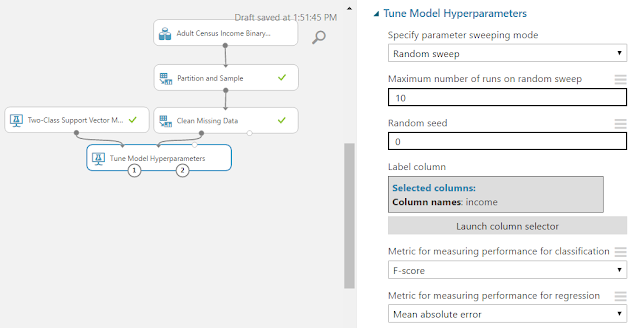Tune Model Hyperparameters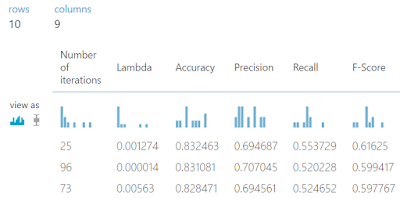Tune Model Hyperparameters (Visualize)
As you can see, the best model has 25 iterations with a Lambda of .001274.  Let's plug that into our Two-Class Support Vector Machine algorithm and move on to Cross-Validation.Cross Validate ModelContingency Table (Two-Class Averaged Perceptron)Contingency Table (Two-Class Boosted Decision Tree)Contingency Table (Two-Class Logistic Regression)Contingency Table (Two-Class Support Vector Machine)
As you can see, the Two-Class Support Vector Machine approach has about the same amount of True positives for "income = '<=50k'" as the rest of the models.  However, the number of true positives for "income = '>50k'" is significantly less than that of the Two-Class Boosted Decision Tree.  Therefore, using accuracy alone, we can say that the Two-Class Boosted Decision Tree model is the best model for this data.

We've mentioned a couple of times that there are more ways to measure "goodness" of a model besides Accuracy.  In order to look at these, let's examine another module called "Evaluate Model".Evaluate Model
There are no parameters to set for the "Evaluate Model" module.  All you do is provide it 1 or 2 scored datasets and it will provide a huge amount of information about the "goodness" of those models.  Here's a snippet of what you can find.Roc Curve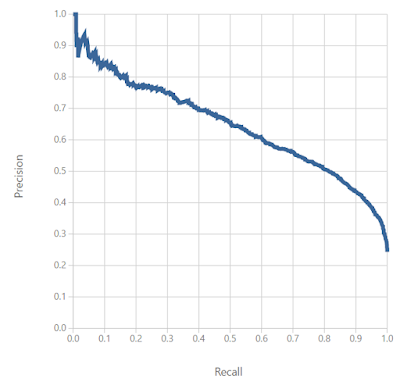Precision/Recall Curve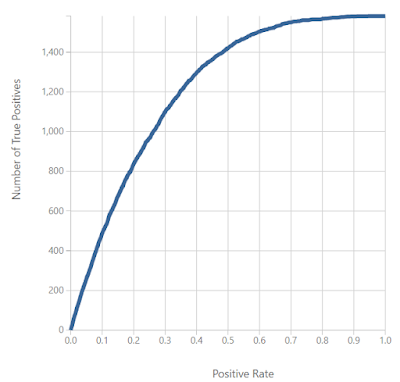Lift Curve
The three charts shown above are the ROC Curve, Precision/Recall Curve, and Lift Curve.  We simply wanted to introduce these concepts to you in this post.  We'll spend a lot more time talking about these metrics in a later post.  Thanks for reading.  We hope you found this informative.